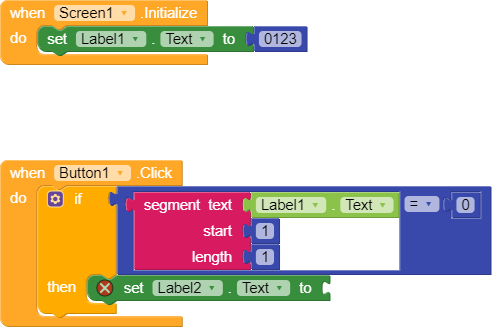# How to remove zero from the number

i have number in label 1 = 0123
now if the first digit of the number is zero then remove zero from the number
IF NUMBER = 0123 THEN RESULT = 123
IF NUMBER = 2345 = THEN RESULT = 23451 Like

Try to multiply by 1
Taifun

2 Likes

This topic was automatically closed 30 days after the last reply. New replies are no longer allowed.